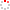NSSC OpenIR  > 空间科学部
 Data completion with Hilbert transform over plane rectangle: Technique renovation for the Grad-Shafranov reconstruction Li, H. J.; Li, C. Y.; Feng, X. S.; Xiang, J.; Huang, Y. Y.; Zhou, S. D.; Xiang, J; Zhou, SD (reprint author), PLA Univ Sci & Technol, Coll Meteorol & Oceanog, Nanjing, Jiangsu, Peoples R China.; Feng, XS (reprint author), Chinese Acad Sci, SIGMA Weather Grp, State Key Lab Space Weather, Ctr Space Sci & Appl Res, Beijing, Peoples R China. Department 空间科学部 Source Publication JOURNAL OF GEOPHYSICAL RESEARCH-SPACE PHYSICS2017 Volume 122Issue:4Pages:3949-3960 ISSN 2169-9380 Language 英语 Abstract Hilbert transforms (HT) have first been used to build the essential technique of Grad-Shafranov (GS) reconstruction by Li et al. (2013), where the problem of ill posedness in GS reconstruction has been thoroughly investigated. In this study, we present an extended Hilbert transform (EHT) over the plane rectangle. In contrast to previous one (HT over the unit circular region), corner singularities are introduced into these new formulae. It is confronted by problems like the integral with both endpoint singularities, and the semiinfinite integral with one endpoint singularity, as these EHT formulae are used to rebuild the essential technique of GS reconstruction. Two additional mathematic tools are adopted in this study. First, high-accuracy quadrature schemes are constructed for those improper integrals based on the double exponential (DE) transformations. Benchmark testing with the analytic solutions on a rectangular boundary has shown the efficiency and robustness of the EHT formulae. Second, the data completion or the inverse boundary value problem is solved with the help of a truncated Chebyshev series, which approximates the unknown boundary gradients in very high efficiency under the only assumption that they are Lipschitz continuous on each side of the rectangle. Combining the introduced EHT formulae and the two needed mathematic tools, the essential technique of GS reconstruction is formulated into a linear system of Fredholm equations of the first kind. Then a three-parameter Tikhonov regularization scheme is developed to deal with the ill-posed linear operators appearing in the discretized linear system. This new approach for data completion over the plane rectangle is benchmarked with the analytic solutions. Numerical experiments highlight the efficiency and robustness of the proposed method. Plain Language Summary The ill posedness for the essential technique of GS reconstruction are solved in this study. The Hilbert transform over plane circle are extended (EHT) to the one over plane rectangle. New data completion approach is built with this new EHT formulae, and a new three-parameter regularization is developed to get a stable solution from the first-kind Fredholm equation. Numerical experiments are also carried out with the analytic solutions. Bench case testing results from the forward computation and its reversion highlights its efficiency and accuracy. Indexed By SCI Document Type 期刊论文 Identifier http://ir.nssc.ac.cn/handle/122/5989 Collection 空间科学部 Corresponding Author Xiang, J; Zhou, SD (reprint author), PLA Univ Sci & Technol, Coll Meteorol & Oceanog, Nanjing, Jiangsu, Peoples R China.; Feng, XS (reprint author), Chinese Acad Sci, SIGMA Weather Grp, State Key Lab Space Weather, Ctr Space Sci & Appl Res, Beijing, Peoples R China. Recommended CitationGB/T 7714 Li, H. J.,Li, C. Y.,Feng, X. S.,et al. Data completion with Hilbert transform over plane rectangle: Technique renovation for the Grad-Shafranov reconstruction[J]. JOURNAL OF GEOPHYSICAL RESEARCH-SPACE PHYSICS,2017,122(4):3949-3960. APA Li, H. J..,Li, C. Y..,Feng, X. S..,Xiang, J..,Huang, Y. Y..,...&Feng, XS .(2017).Data completion with Hilbert transform over plane rectangle: Technique renovation for the Grad-Shafranov reconstruction.JOURNAL OF GEOPHYSICAL RESEARCH-SPACE PHYSICS,122(4),3949-3960. MLA Li, H. J.,et al."Data completion with Hilbert transform over plane rectangle: Technique renovation for the Grad-Shafranov reconstruction".JOURNAL OF GEOPHYSICAL RESEARCH-SPACE PHYSICS 122.4(2017):3949-3960.